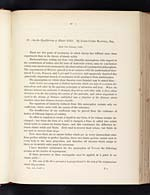# James Clerk Maxwell (1831-1879)

## On the equilibrium of elastic solids

(   87     )

IV.—On the Equilibrium of Elastic Solids. By JAMES CLERK MAXWELL, Esq.

There are few parts of mechanics in which theory has differed more from
experiment than in the theory of elastic solids.

Mathematicians, setting out from very plausible assumptions with respect to
the constitution of bodies, and the laws of molecular action, came to conclusions
which were shewn to be erroneous by the observations of experimental philosophers.
The experiments of ŒRSTED proved to be at variance with the mathematical theo-
ries of NAVIER, POISSON, and LAMÉ and CLAPEYRON, and apparently deprived this
practically important branch of mechanics of all assistance from mathematics.

The assumption on which these theories were founded may be stated thus :—

Solid bodies are composed of distinct molecules, which are kept at a certain dis-
tance from each other by the opposing principles of attraction and heat. When the
distance between two molecules is changed, they act on each other with a force whose
direction is in the line joining the centres of the molecules, and whose magnitude is
equal to the change of distance multiplied into a function of the distance which
vanishes when that distance becomes sensible.

The equations of elasticity deduced from this assumption contain only one
coefficient, which varies with the nature of the substance.

The insufficiency of one coefficient may be proved from the existence of
bodies of different degrees of solidity.

No effort is required to retain a liquid in any form, if its volume remain un-
changed; but when the form of a solid is changed, a force is called into action
which tends to restore its former figure; and this constitutes the difference be-
tween elastic solids and fluids. Both tend to recover their volume, but fluids do
not tend to recover their shape.

Now, since there are in nature bodies which are in every intermediate state
from perfect solidity to perfect liquidity, these two elastic powers cannot exist in
every body in the same proportion, and therefore all theories which assign to
them an invariable ratio must be erroneous.

I have therefore substituted for the assumption of NAVIER the following
axioms as the results of experiments.

If three pressures in three rectangular axes be applied at a point in an
elastic solid,—

1. The sum of the three pressures is proportional to the sum of the compressions
which they produce.

VOL. XX. PART I.

2 AView larger image